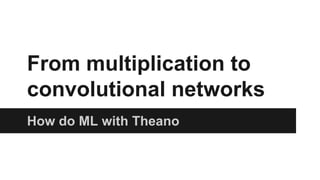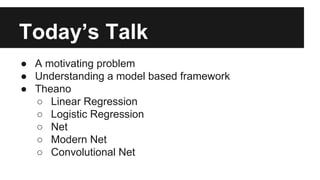Diese Präsentation wurde erfolgreich gemeldet.

# Introduction to Deep Learning with Python

Anzeige
Anzeige
Anzeige
Anzeige
Anzeige
Anzeige
Anzeige
Anzeige
Anzeige
Anzeige
AnzeigeYouTube-Videos werden auf SlideShare nicht mehr unterstützt.×

1 von 82 Anzeige

# Introduction to Deep Learning with Python

A presentation by Alec Radford, Head of Research at indico Data Solutions, on deep learning with Python's Theano library.

The emphasis of the presentation is high performance computing, natural language processing (using recurrent neural nets), and large scale learning with GPUs.

Video of the talk available here: https://www.youtube.com/watch?v=S75EdAcXHKk

A presentation by Alec Radford, Head of Research at indico Data Solutions, on deep learning with Python's Theano library.

The emphasis of the presentation is high performance computing, natural language processing (using recurrent neural nets), and large scale learning with GPUs.

Video of the talk available here: https://www.youtube.com/watch?v=S75EdAcXHKk

Anzeige
Anzeige

### Introduction to Deep Learning with Python

1. 1. From multiplication to convolutional networks How do ML with Theano
2. 2. Today’s Talk ● A motivating problem ● Understanding a model based framework ● Theano ○ Linear Regression ○ Logistic Regression ○ Net ○ Modern Net ○ Convolutional Net
3. 3. Follow along Tutorial code at: https://github.com/Newmu/Theano-Tutorials Data at: http://yann.lecun.com/exdb/mnist/ Slides at: http://goo.gl/vuBQfe
4. 4. A motivating problem How do we program a computer to recognize a picture of a handwritten digit as a 0-9? What could we do?
5. 5. A dataset - MNIST What if we have 60,000 of these images and their label? X = images Y = labels X = (60000 x 784) #matrix (list of lists) Y = (60000) #vector (list) Given X as input, predict Y
6. 6. An idea For each image, find the “most similar” image and guess that as the label.
7. 7. An idea For each image, find the “most similar” image and guess that as the label. KNearestNeighbors ~95% accuracy
8. 8. Trying things Make some functions computing relevant information for solving the problem
9. 9. What we can code Make some functions computing relevant information for solving the problem feature engineering
10. 10. What we can code Hard coded rules are brittle and often aren’t obvious or apparent for many problems.
11. 11. Model A Machine Learning Framework 8 Inputs Computation Outputs
12. 12. from arXiv:1409.4842v1 [cs.CV] 17 Sep 2014 A … model? - GoogLeNet
13. 13. 3 mult Input Computation Output A very simple model by x 12
14. 14. Theano intro
15. 15. Theano intro imports
16. 16. Theano intro imports theano symbolic variable initialization
17. 17. Theano intro imports theano symbolic variable initialization our model
18. 18. Theano intro imports theano symbolic variable initialization our model compiling to a python function
19. 19. Theano intro imports theano symbolic variable initialization our model compiling to a python function usage
20. 20. Theano
21. 21. Theano imports
22. 22. Theano imports training data generation
23. 23. Theano imports training data generation symbolic variable initialization
24. 24. Theano imports training data generation symbolic variable initialization our model
25. 25. Theano imports training data generation symbolic variable initialization our model model parameter initialization
26. 26. Theano imports training data generation symbolic variable initialization our model model parameter initialization metric to be optimized by model
27. 27. Theano imports training data generation symbolic variable initialization our model model parameter initialization metric to be optimized by model learning signal for parameter(s)
28. 28. Theano imports training data generation symbolic variable initialization our model model parameter initialization metric to be optimized by model learning signal for parameter(s) how to change parameter based on learning signal
29. 29. Theano imports training data generation symbolic variable initialization our model model parameter initialization metric to be optimized by model learning signal for parameter(s) how to change parameter based on learning signal compiling to a python function
30. 30. Theano imports training data generation symbolic variable initialization our model model parameter initialization metric to be optimized by model learning signal for parameter(s) how to change parameter based on learning signal compiling to a python function iterate through data 100 times and train model on each example of input, output pairs
31. 31. Theano doing its thing
32. 32. Zero One Two Three Four Five Six Seven Eight Nine 0.1 0. 0. 0.1 0. 0. 0. 0. 0.7 0.1 Logistic Regression softmax(X) T.dot(X, w)
33. 33. Back to Theano
34. 34. Back to Theano convert to correct dtype
35. 35. Back to Theano convert to correct dtype initialize model parameters
36. 36. Back to Theano convert to correct dtype initialize model parameters our model in matrix format
37. 37. Back to Theano convert to correct dtype initialize model parameters our model in matrix format loading data matrices
38. 38. Back to Theano convert to correct dtype initialize model parameters our model in matrix format loading data matrices now matrix types
39. 39. Back to Theano convert to correct dtype initialize model parameters our model in matrix format loading data matrices now matrix types probability outputs and maxima predictions
40. 40. Back to Theano convert to correct dtype initialize model parameters our model in matrix format loading data matrices now matrix types probability outputs and maxima predictions classification metric to optimize
41. 41. Back to Theano convert to correct dtype initialize model parameters our model in matrix format loading data matrices now matrix types probability outputs and maxima predictions classification metric to optimize compile prediction function
42. 42. Back to Theano convert to correct dtype initialize model parameters our model in matrix format loading data matrices now matrix types probability outputs and maxima predictions classification metric to optimize compile prediction function train on mini-batches of 128 examples
43. 43. 0 1 2 3 4 5 6 7 8 9 What it learns
44. 44. 0 1 2 3 4 5 6 7 8 9 What it learns Test Accuracy: 92.5%
45. 45. Zero One Two Three Four Five Six Seven Eight Nine 0.0 0. 0. 0.1 0. 0. 0. 0. 0.9 0. y = softmax(T.dot(h, wo)) h = T.nnet.sigmoid(T.dot(X, wh)) An “old” net (circa 2000)
46. 46. A “old” net in Theano
47. 47. A “old” net in Theano generalize to compute gradient descent on all model parameters
48. 48. 2D moons dataset courtesy of scikit-learn Understanding SGD
49. 49. A “old” net in Theano generalize to compute gradient descent on all model parameters 2 layers of computation input -> hidden (sigmoid) hidden -> output (softmax)
50. 50. Understanding Sigmoid Units
51. 51. A “old” net in Theano generalize to compute gradient descent on all model parameters 2 layers of computation input -> hidden (sigmoid) hidden -> output (softmax) initialize both weight matrices
52. 52. A “old” net in Theano generalize to compute gradient descent on all model parameters 2 layers of computation input -> hidden (sigmoid) hidden -> output (softmax) initialize both weight matrices updated version of updates
53. 53. What an “old” net learns Test Accuracy: 98.4%
54. 54. Zero One Two Three Four Five Six Seven Eight Nine 0.0 0. 0. 0.1 0. 0. 0. 0. 0.9 0. y = softmax(T.dot(h2, wo)) h2 = rectify(T.dot(h, wh)) h = rectify(T.dot(X, wh)) Noise Noise Noise (or augmentation) A “modern” net - 2012+
55. 55. A “modern” net in Theano
56. 56. rectifier A “modern” net in Theano
57. 57. Understanding rectifier units
58. 58. rectifier numerically stable softmax A “modern” net in Theano
59. 59. rectifier numerically stable softmax a running average of the magnitude of the gradient A “modern” net in Theano
60. 60. rectifier numerically stable softmax a running average of the magnitude of the gradient scale the gradient based on running average A “modern” net in Theano
61. 61. 2D moons dataset courtesy of scikit-learn Understanding RMSprop
62. 62. rectifier numerically stable softmax a running average of the magnitude of the gradient scale the gradient based on running average A “modern” net in Theano randomly drop values and scale rest
63. 63. rectifier numerically stable softmax a running average of the magnitude of the gradient scale the gradient based on running average A “modern” net in Theano randomly drop values and scale rest Noise injected into model rectifiers now used 2 hidden layers
64. 64. What a “modern” net learns Test Accuracy: 99.0%
65. 65. Quantifying the difference
66. 66. What a “modern” net is doing
67. 67. from deeplearning.net Convolutional Networks
68. 68. A convolutional network in Theano
69. 69. a “block” of computation conv -> activate -> pool -> noise A convolutional network in Theano
70. 70. a “block” of computation conv -> activate -> pool -> noise convert from 4tensor to normal matrix A convolutional network in Theano
71. 71. a “block” of computation conv -> activate -> pool -> noise convert from 4tensor to normal matrix reshape into conv 4tensor (b, c, 0, 1) format A convolutional network in Theano
72. 72. a “block” of computation conv -> activate -> pool -> noise convert from 4tensor to normal matrix reshape into conv 4tensor (b, c, 0, 1) format now 4tensor for conv instead of matrix A convolutional network in Theano
73. 73. a “block” of computation conv -> activate -> pool -> noise convert from 4tensor to normal matrix reshape into conv 4tensor (b, c, 0, 1) format now 4tensor for conv instead of matrix conv weights (n_kernels, n_channels, kernel_w, kerbel_h) A convolutional network in Theano
74. 74. a “block” of computation conv -> activate -> pool -> noise convert from 4tensor to normal matrix reshape into conv 4tensor (b, c, 0, 1) format now 4tensor for conv instead of matrix conv weights (n_kernels, n_channels, kernel_w, kerbel_h) highest conv layer has 128 filters and a 3x3 grid of responses A convolutional network in Theano
75. 75. a “block” of computation conv -> activate -> pool -> noise convert from 4tensor to normal matrix reshape into conv 4tensor (b, c, 0, 1) format now 4tensor for conv instead of matrix conv weights (n_kernels, n_channels, kernel_w, kerbel_h) highest conv layer has 128 filters and a 3x3 grid of responses A convolutional network in Theano noise during training
76. 76. a “block” of computation conv -> activate -> pool -> noise convert from 4tensor to normal matrix reshape into conv 4tensor (b, c, 0, 1) format now 4tensor for conv instead of matrix conv weights (n_kernels, n_channels, kernel_w, kerbel_h) highest conv layer has 128 filters and a 3x3 grid of responses A convolutional network in Theano noise during training no noise for prediction
77. 77. Test Accuracy: 99.5% What a convolutional network learns
78. 78. Takeaways ● A few tricks are needed to get good results ○ Noise important for regularization ○ Rectifiers for faster, better, learning ○ Don’t use SGD - lots of cheap simple improvements ● Models need room to compute. ● If your data has structure, your model should respect it.
79. 79. Resources ● More in-depth theano tutorials ○ http://www.deeplearning.net/tutorial/ ● Theano docs ○ http://www.deeplearning.net/software/theano/library/ ● Community ○ http://www.reddit.com/r/machinelearning
80. 80. A plug Keep up to date with indico: https://indico1.typeform.com/to/DgN5SP
81. 81. Questions?